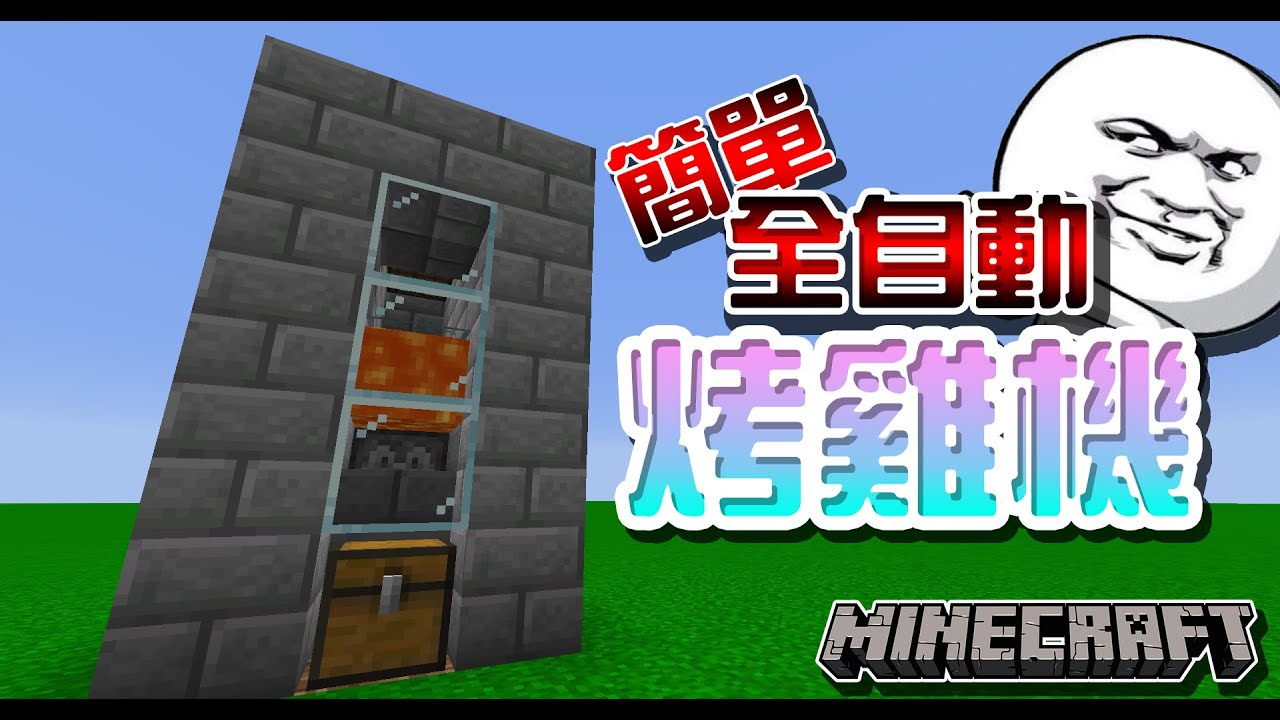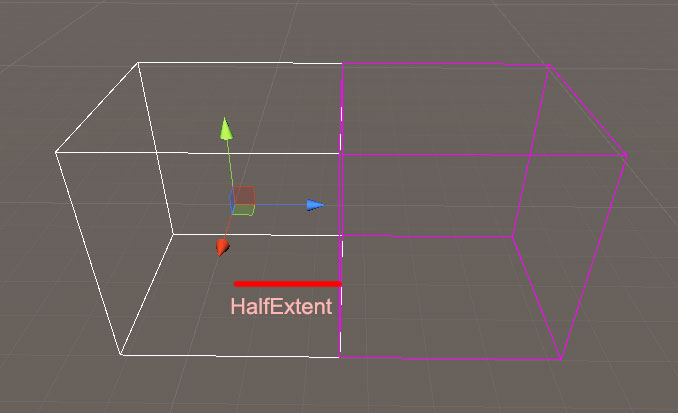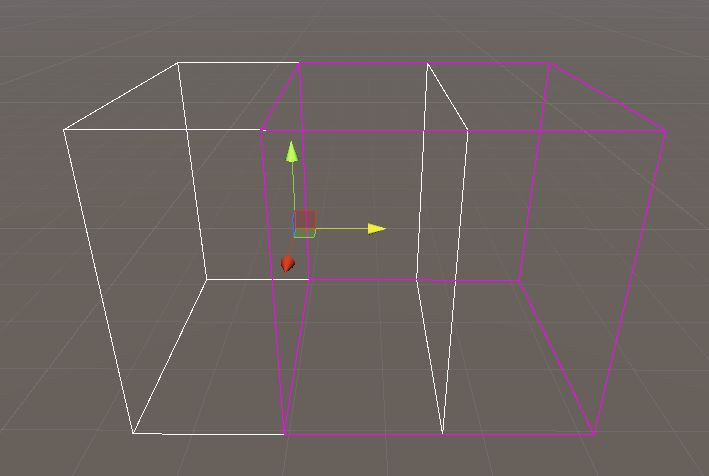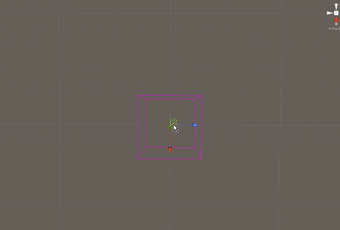# 正規化座標 Structured GridMinicraft 畫面示意圖.

```public Bounds GetCell(Vector3 point, float halfExtent)
{
float size = halfExtent * 2f;
Vector3 size3D = new Vector3(size, size, size);
point = new Vector3(
Mathf.Sign(point.x) * (Mathf.Abs(point.x) + halfExtent),
Mathf.Sign(point.y) * (Mathf.Abs(point.y) + halfExtent),
Mathf.Sign(point.z) * (Mathf.Abs(point.z) + halfExtent));
Vector3 remainder = new Vector3(
point.x % size,
point.y % size,
point.z % size);
Vector3 center = point - remainder;
return new Bounds(center, size3D);
}```

point.x – (point.x % size)

= 1.5f – (1.5f mod 1f)
= 1.5f – 0.5f
= 1ffinal result.